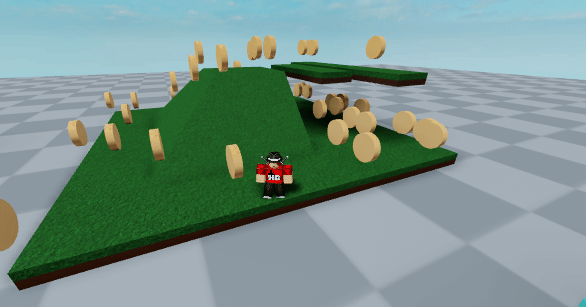# How Would I Make My Coin Script Spawn Random Coins Within A certain Radius?

I want my coins to spawn randomly around a map but only in a certain radius from the center point.
I’ve tried to make it work but I’m not good at this stuff so any help would be appreciatedScript below

``````local Coins = game.Workspace.Coins
local CoinTemplate = script.Coin

local SpawnCoins = function()

if game.ServerStorage.Values.RoundActive.Value == true then
if #game.Workspace.Coins:GetChildren() < 25 then
if game.Workspace:FindFirstChild("Map") then
local Center = game.Workspace:FindFirstChild("Map").CoinParams.Center
local CoinClone = CoinTemplate:Clone()

local y = Center.Position.Y
CoinClone.Position = Vector3.new(x,y,z)
CoinClone.Parent = Coins
end

end
end

end
``````Wow! didn’t know this existed, thanks